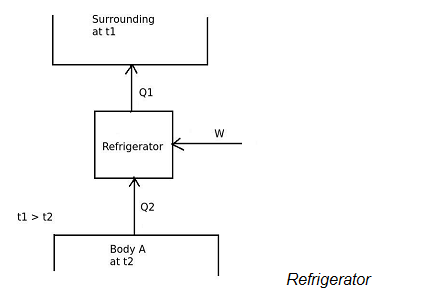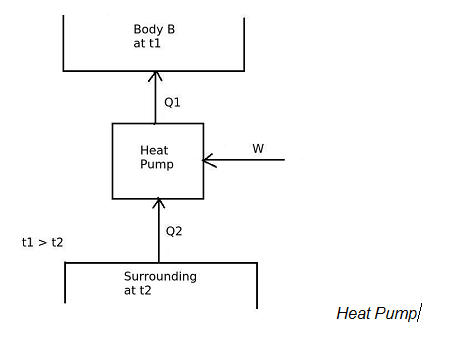# Which among the following is correct relation between COP of heat pump and COP of refrigerator ?

## Which among the following is correct relation between COP of heat pump and COP of refrigerator ?

a. [COP]H.P. = 1 + [COP]ref
b. [COP]H.P. = 1 – [COP]ref
c. [COP]H.P. = [COP]ref
d. none of the above

Correct Answer : a. [COP]H.P. = 1 + [COP]ref

Explanation :
The COP of a device, operating in a cycle is given by,
[COP] = Desired effect / Work input
In refrigerator,the desired effect is to maintain temperature of the body A lower than the surrounding temperature.
Therefore, A refrigerator is a device, which operates in a cycle and maintains the temperature a particular body lower than the surrounding temperature.
[COP]ref = Desired effect / Work input
[COP]ref = Q2 / W

[COP]H.P
And in heat pump,the desired effect is to maintain temperature of the body B more than the surrounding temperature.
Therefore,
[COP]H.P. = Desired effect / Work input
[COP]H.P = Q1 / W

and the work input is given by,
W = Q1 – Q2

From the equations of [COP]ref and [COP]H.P ,
[COP]H.P. = 1 + [COP]ref